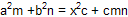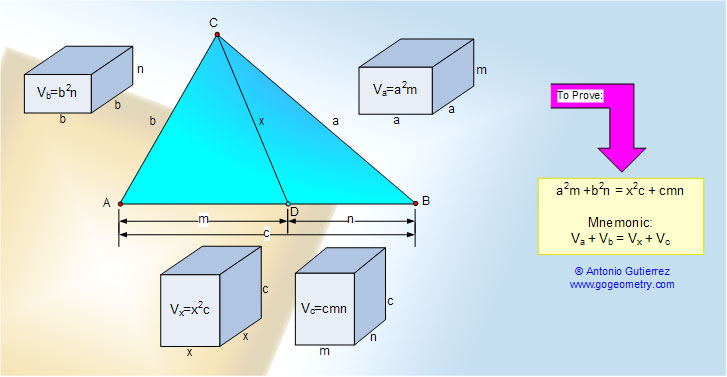# Online Geometry Problem 805: Stewart's Theorem, Triangle, Sides, Cevian, Metric Relations, Measurement. Level: High School, SAT Prep, College, Mathematics Education

 Let be given a triangle ABC and a point D on AB such that m = AD, n = BD, and x = CD. Then Stewart's theorem, also called Apollonius' theorem, states that:.Home | Search | Geometry | Problems | All Problems | Open Problems | Visual Index | 801-810 | Triangles | Right Triangle Pythagoras Mind Map | Email | Solution / comment | By Antonio Gutierrez